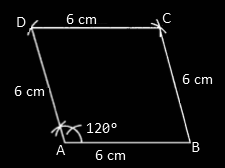# RBSE Maths Class 9 Chapter 9: Important Questions and Solutions

RBSE Maths Chapter 9 – Quadrilateral Class 9 Important questions and solutions are available here. The important questions and solutions of Chapter 9, provided at BYJU’S, are prepared by our expert faculty with step by step explanations. Also, RBSE Class 9 solutions are provided here to help the students understand all the concepts clearly.

Chapter 9 of the RBSE Class 9 Maths contains major important concepts related to quadrilaterals. Important questions for all the exercises are given here, which cover construction of different quadrilaterals, namely parallelogram, rhombus, square, rectangle and trapezium. After practicing these important questions, students will be able to prove certain statements with a combination of two or more quadrilaterals and their properties.

## RBSE Maths Chapter 9: Exercise 9.1 Textbook Important Questions and Solutions

Question 1: Diagonals AC and BD of a parallelogram ABCD intersect each other at point O, where OA = 3 cm and OD = 2 cm. Find the lengths of AC and BD.

Solution:

Given that,

OA = 3 cm and OD = 2 cm in parallelogram ABCD.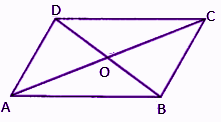We know that diagonals of a parallelogram bisect each other.

AC = 2OA = 2 x 3 cm = 6 cm

Similarly,

BD = 2OD = 2 x 2 cm = 4 cm

Therefore, AC = 6 cm and BD = 4cm.

Question 2: The diagonals of a parallelogram are perpendicular to each other. Is this statement true? Give reason in support of your answer.

Solution:

No, the diagonals of a parallelogram are not perpendicular to each other but they bisect each other, as shown in the below figure.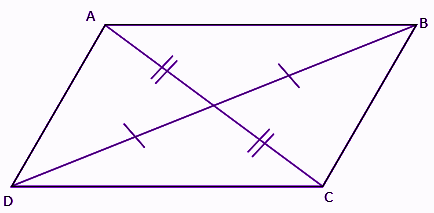Question 3: State whether the angles 110°, 80°, 70°, and 95° are angles of a quadrilateral. Why and why not?

Solution:

We know that the sum of all the interior angles of a quadrilateral = 360°

From the given,

110° + 80° +70° + 95° = 355°

Here, the sum of all the angles is not equal to 360°.

Hence, they are not the angles of a quadrilateral.

Solution:

No, if all the angles will be obtuse (that means greater than 90°) then the sum of angles of a quadrilateral will exceed 360°.

For example:

91° + 92° + 100° + 95° = 378° > 360°

Question 5: One angle of a quadrilateral is 108° and the other three angles are equal. Find the measure of each of the three equal angles.

Solution:

Let x be the measure of each of the three equal angles.

By the angle sum property of a quadrilateral,

108° + x + x + x = 360°

3x + 108° = 360°

3x = 360° – 108°

3x = 252°

x = 252°/3 = 84°

Therefore, the measure of the equal angle is 84°.

Question 6: ABCD is a trapezium in which AB || DC and ∠A = ∠B = 45°. Find ∠C and ∠D of this trapezium.

Solution:

Given,

AB || DC

∠A = ∠B = 45°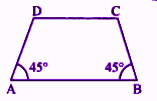We know that the sum of the interior angles on the same side of a transversal is always 180°.

∠A + ∠D = 180°

45° + ∠D = 180°

⇒ ∠D = 135°

Similarly,

∠B + ∠C = 180°

45° + ∠C = 180°

∠C = 135°

Question 7: The angle between two altitudes drawn from the vertex of an obtuse angle to the opposite sides of a parallelogram is 60°. Find all the angles of this parallelogram.

Solution:

Let ABCD be the parallelogram in which ∠D is obtuse.

Through the vertex D altitudes, DE and DF are drawn.

According to the given,

∠DEF = 60°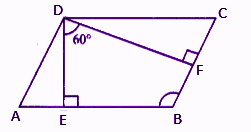∠EDF + ∠DEB + ∠EBF + ∠DFB = 360°

(by the angle sum property of a quadrilateral)

60° + 90° + ∠EBF + 90° = 360°

∠EBF = 360° – 240°

∠EBF = 120°

⇒∠ABC = 120°

(opposite angles of a parallelogram are equal)

Also,

(adjacent angles of a parallelogram are supplementary)

(opposite angles of a parallelogram)

Hence, angles of the parallelogram are 120°, 60°, 120° and 60°.

Question 8: Points E and F lie on diagonal AC of a parallelogram ABCD such that AE = CF. Show that BFDE is a parallelogram.

Solution:

From the given,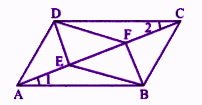In ∆ABE and ∆CDF,

AB = DC

(opposite sides of a parallelogram)

AE = CF (given)

and ∠1 = ∠2 (alternate interior angles)

By SAS congruence criterion,

∆ABE ≅ ∆CDF

⇒ BE = BF ….(i) (by CPCT)

Similarly,

∆AED ≅ ∆BFC (by SAS congruence criterion)

DE = BF ….(ii) (by CPCT)

From (i) and (ii),

BFDE is a parallelogram.

Question 9: In figure, ABCD is a parallelogram. AQ and CP are the bisectors of ∠A and ∠C, respectively. Prove the APCQ is a parallelogram.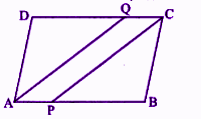Solution:

Given,

ABCD is a parallelogram.

AQ and CP are the bisectors of ∠A and ∠C, respectively.

In parallelogram ABCD,

∠A = ∠C (opposite angles of a parallelogram are equal)

(1/2) ∠A = (1/2) ∠C

⇒ ∠PAQ = ∠PCQ (since AQ bisect ∠A and PC bisect ∠C)

Therefore, AB || CD

⇒ AP || CQ

That means, pairs of opposite sides are parallel.

APCQ is a parallelogram.

Hence, proved.

Question 10: In the figure, ABCD and AFEB are parallelograms. Prove that CDFE is a parallelogram.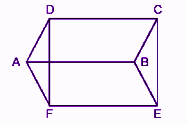Solution:

ABCD is a parallelogram

AB = DC

and AB || DC ….(i)

Reason: Opposite sides of a parallelogram are equal and parallel. Again ABEF is a parallelogram

⇒ AB = FE

and AB || FE ….(ii)

From (i) and (ii),

DC = FE and DC || FE

Therefore, CDFE is a parallelogram.

Hence proved.

### RBSE Maths Chapter 9: Exercise 9.2 Textbook Important Questions and Solutions

Question 11: In the figure, ABCD and AEFG are two parallelograms. If ∠C = 55°, then determine ∠F.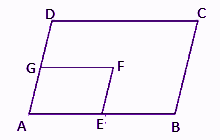Solution:

Given,

ABCD is a parallelogram.

⇒ ∠A = ∠C = 55° (opposite angles are equal)

Also given that, AEFG is a parallelogram.

Therefore, ∠A = ∠F = 55°.

Question 12: The diagonals of a quadrilateral ABCD bisect each other. If ∠A = 35°, then determine ∠B.

Solution:

Given that,

Diagonals of a quadrilateral bisect each other.

That means ABCD is a parallelogram.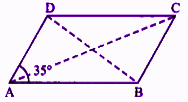We know that the sum of interior angles on the same side of a transversal is 180°.

∠A + ∠B = 180°

35° + ∠B = 180°

∠B = 180° – 35°

∠B = 145°

Question 13: Opposite angles of a quadrilateral ABCD are equal. If AB = 4 cm then determine CD.

Solution:

Given that,

Opposite angles of a quadrilateral ABCD are equal.

That means, the given quadrilateral is a parallelogram.

We know that the opposite sides of a parallelogram are equal.

Therefore, CD = AB = 4 cm

Question 14: ABCD is a rhombus in which altitude drawn from D on AB, bisects AB. Find the angles of the rhombus.

Solution:

Let x be the side of the rhombus ABCD.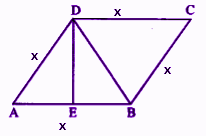In right triangle AED,

By Pythagoras theorem,

x2 = (x/2)2 + DE2

DE2 = x2 – x2/4

DE2 = (4x2 – x2)/4

DE2 = 3x2/4

DE = √(3x2/4)

DE = (√3/2) x

Similarly, in right triangle DEB,

By Pythagoras theorem, we can get the value of DB.

DB = x

Thus, ABD is an equilateral triangle.

∠A = 60°

In rhombus ABCD,

⇒ ∠A + ∠D = 180°

⇒ ∠D = 120°

Similarly, ∠C = 60°, ∠D = 120°

Therefore, the angles of the rhombus are ∠A = 60°, ∠B = 120°, ∠C = 60° and ∠D = 120°.

Question 15: In a triangle ABC, lines RQ, PR and QP are respectively drawn parallel to lines BC, CA and AB passing through A, B and C as shown in the figure. Show that BC = 1/2 QR.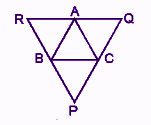Solution:

From the given,

PQ || AB, PR || AC and RQ || BC.

BR || CA and BC || RA (from the given figure)

Therefore, BCAR is a parallelogram.

⇒ BC = AR ….(i)

BC || AQ and AB || QC (from the given figure)

Therefore, BCQA is a parallelogram.

⇒ BC = AQ ….(ii)

BC + BC = AR + AQ

2BC = RQ

BC = 1/2 QR

Question 16: D, E and F are respectively the mid-points of sides BC, CA and AB of an equilateral triangle ABC. Show that ∆DEF is also an equilateral triangle.

Solution:

Given,

D, E and F are mid-points of sides BC,CA and AB respectively of an equilateral triangle ABC.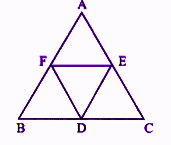Using mid point theorem,

DE ∥ AB and DE = 1/2 AB ….(i)

Also,

FE ∥ BC and FE = 1/2 BC ….(ii)

Similarly,

FD ∥ AC and FD = 1/2 AC ….(iii)

ΔABC is an equilateral triangle. (given)

AB = BC = CA

⇒ 1/2 AB = 1/2 BC = 1/2 CA ….(iv)

From (i), (ii), (iii), and (iv),

DE = FE = FD

Therefore, ΔDEF is an equilateral triangle.

Question 17: Points P and Q are respectively taken on opposite sides AB and CD of a parallelogram ABCD such that AP = CQ (see in the figure). Show that AC and PQ bisect each other.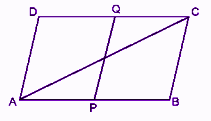Solution:

Given that ABCD is a parallelogram and AP = CQ.

Let R be the point of intersection of AC and PQ.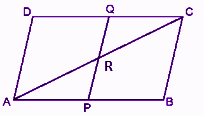∠RAP = ∠RCP (alternate interior angles)

AP = CQ (given)

∠APR = ∠CQR (alternate interior angles)

∴ ∆ARP ≅ ∆CRQ (by ASA congruence criterion)

AR = CR

PR = QR (by CPCT)

Therefore, AC and PQ bisect each other.

Question 18: In ∆ABC, AB = 5 cm, BC = 8 cm and CA = 7 cm. If D and E are mid-points of sides AB and BC respectively, then find the length of DE.

Solution:

Given that,

In ∆ABC, D and E are mid-points of sides AB and BC, respectively.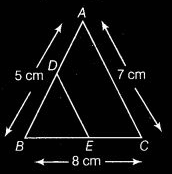Using mid-point theorem,

DE = 1/2 AC

= (1/2) × 7

= 3.5 cm

Question 19: In the figure, it is given that BDEF and FDCE are parallelograms. Can you say that BD = CD? Why and why not?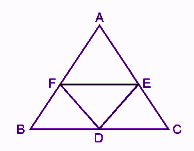Solution:

Given,

BDEF and FDCE are parallelograms.

We know that opposite sides of a parallelogram are equal.

⇒ BD = FE ….(i)

And DC = FE ….(ii)

From (i) and (ii),

BD = CD

Question 20: Prove that a rhombus is obtained by joining the mid-points of the pairs of opposite sides of a rectangle.

Solution:

Let ABCD be the rectangle in which P, Q, R and S are the mid-points of sides AB, BC, CD and DA, respectively.

Join PQ, QR, RS and SP.

Also, join AC.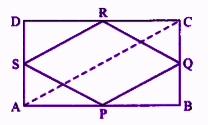In ∆ABC,

P and Q are the mid-point of sides AB and BC, respectively.

Using mid-point theorem,

Therefore, PQ || AC and PQ = 1/2 AC ….(i)

SR || AC and SR = 1/2 AC ….(ii)

From (i) and (ii),

PQ || SR and PQ = SR ….(iii)

Therefore, PQRS is a parallelogram.

ABCD is a rectangle. (given)

⇒ 1/2 AD = 1/2 BC

⇒ AS = BQ ….(iv)

⇒ In triangle APS and triangle BPQ,

AP = BP

(since P is the mid-point of AB)

∠PAS = ∠PBQ = 90°

AS = BQ [ from (iv)]

By SAS congruence criterion,

∆APS ≅ ∆BPQ

PS = PQ ….(v) (by CPCT)

From (iii) and (v),

PQRS is a parallelogram.

PS = PQ

Thus, PQRS is a rhombus.

Hence proved.

### RBSE Maths Chapter 9: Exercise 9.3 Textbook Important Questions and Solutions

Question 21: Construct a quadrilateral ABCD, with AB = 3.5 cm, BC = 4.8 cm, CD = 5.1 cm, AD = 4.4 cm and a diagonal AC = 5.9 cm.

Solution:

Steps of construction:

(i) Draw a line segment AB of length 3.5 cm as the base.

(ii) Taking A as the centre, draw an arc with radius 5.9 cm.

(iii) With B as the centre, draw an arc with radius 4.8 cm, which intersects the previously drawn arc at C.

(iv) Join AC and BC.

(v) Now, by taking C as the centre and draw an arc of radius 5.1 cm and with A as the centre and radius 4.4 cm draw another arc. These arcs intersect each other at D.

Hence, ABCD is the required quadrilateral.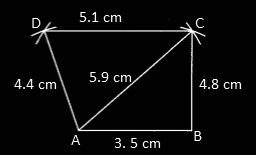Question 22: Construct a quadrilateral ABCD, with AB = 4 cm, BC = 4.5 cm, CD = 3.5 cm, AD = 3 cm and ∠A = 60°.

Solution:

Steps of construction:

(i) Draw a line segment AB of length 4 cm as the base.

(ii) At the point A, draw an angle line of 60°.

(iii) Taking P as the centre, draw an arc of radius 3 cm which intersects the angle line at the point D.

(iv) Now by taking D as the centre, draw an arc of radius 3.5 cm and from B, draw an arc of 4.5 cm which intersects the previous arc at C.

(v) Join CD and BC.

Hence, ABCD is the required quadrilateral.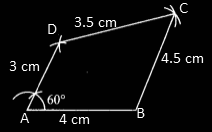Question 23: Construct a quadrilateral ABCD, with AB = 3.5 cm, BC = 3 cm, AD = 2.5 cm, AC = 4.5 cm and BD = 4 cm.

Solution:

Steps of construction:

(i) Draw a line segment AB of length 3.5 cm as the base.

(ii) Taking A and B as centres, draw arcs of radius 4.5 cm and 4 cm, respectively.

(iii) Also, taking B as the centre, draw an arc of radius 3 cm which intersects the previously drawn arc of radius 4.5 cm at C.

(iv) Now, from A, draw an arc of 2.5 cm which intersects the previously drawn arc of 4 cm at D.

(v) Join AD, BC, BD and CD.

Hence, ABCD is the required quadrilateral.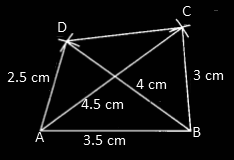Question 24: Construct a quadrilateral ABCD, with AB = BC = 3 cm, AD = 5 cm, ∠A = 90° and ∠B = 120°.

Solution:

Steps of construction:

(i) Draw a line segment AB of length 3 cm as the base.

(ii) At A draw an angle line of 90°.

(iii) At B draw an angle line of 120°.

(iv) Taking A and B as centres and radius 5 cm and 3 cm, draw arcs which cut angle lines of 90° and 120° at D and C, respectively.

(v) Join CD.

Hence, ABCD is the required quadrilateral.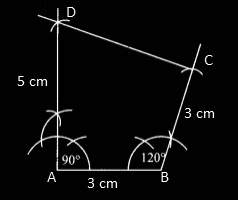Question 25: Construct a quadrilateral PQRS, with PQ = 3 cm, QR = 3.5 cm, ∠Q = 90°, ∠P = 105°, and ∠R = 120°.

Solution:

Steps of construction:

(i) Draw a line segment PQ = 3 cm as base.

(ii) At the point P, draw a ray XP such that ∠QPX = 105°.

(iii) At the point Q, draw a ray QZ such that ∠PQZ = 90°.

(iv) Taking Q as the centre, draw an arc of radius 3.5 cm which intersects the ray QZ at R.

(iv) At the point R, draw an angle line of 120° which cuts PX at S.

Hence, PQRS is the required quadrilateral.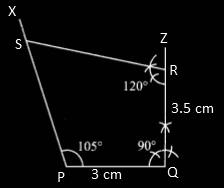### RBSE Maths Chapter 9: Exercise 9.4 Textbook Important Questions and Solutions

Question 26: Construct a parallelogram ABCD in which AB = 4.7 cm, BC = 3.5 cm and AC = 7 cm.

Solution:

Steps of construction:

(i) Draw a line segment AB of length 4.7 cm as the base.

(ii) With centre A, draw arcs of radii 3.5 cm and 7 cm, respectively.

(iii) Taking B as the centre, draw an arc of 3.5 cm which will intersect the previous arc (with radius 3.5 cm) at C.

(iv) Again by taking C as the centre, draw an arc of 4.7 cm which intersects the previous arc (with radius 3.5 cm) at D.

(v) Join AC, AD, BC and CD.

Hence, ABCD is the required parallelogram.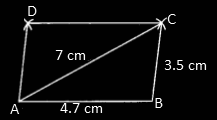Question 27: Construct a parallelogram ABCD whose two sides are 4.6 cm and 3 cm respectively and angle between them is 60°.

Solution:

Steps of construction:

(i) Draw a line segment AB of length 4.6 cm as the base.

(ii) With A as the centre, draw an angle line of 60°.

(iii) Taking A as the centre, draw an arc of 3 cm which intersects the angle line at D.

(iv) Now, by taking D and B as centres draw arcs of 4.6 cm and 3 cm which intersect each other at C.

Hence, ABCD is the required parallelogram.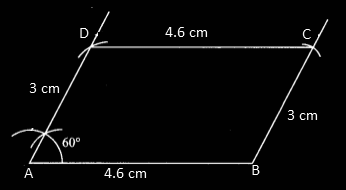Question 28: Construct a rectangle ABCD in which AB = 6 cm and diagonal AC = 10 cm.

Solution:

Steps of construction:

(i) Draw a line segment AB of length 6 cm.

(ii) At point B, draw an angle line of 90°.

(iii) With A as the centre, draw an arc of radius 10 cm which cuts the angle line at C.

(iv) Taking C as the centre, draw an arc of 6 cm.

(v) Again with A as the centre and radius equal to BC, draw an arc which cuts the previous arc at D.

Hence, ABCD is the required rectangle.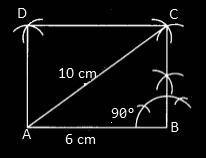Question 29: Construct a rhombus ABCD whose diagonals AC = 7 cm and BD = 5 cm.

Solution:

Steps of construction:

(i) Draw a line segment AC of length 7 cm as the base (one of the diagonals).

(ii) Draw the perpendicular bisector of AC which intersects AC at O.

(iii) Taking O as the centre, draw arcs with radius 2.5 cm above and below the line segment AC.

(iv) These arcs intersect the bisector line at D (above AC) and at B (below AC).

(v) Join AD, CD, AB and BC.

Hence, ABCD is the required rhombus.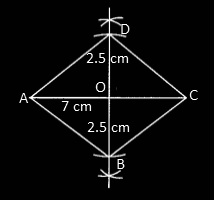Question 30: Construct a square ABCD whose diagonal is 6 cm.

Solution:

Steps of construction:

(i) Draw a line segment BD of length 6 cm as the base (diagonal).

(ii) Draw the perpendicular bisector of DB which intersects DB at O.

(iii) Taking O as the centre, draw arcs with radius 3 cm above and below the line segment DB.

(iv) These arcs intersect the bisector line at C (above DB) and at A (below DB).

(v) Join AB, AD, BC and BD.

Hence, ABCD is the required square.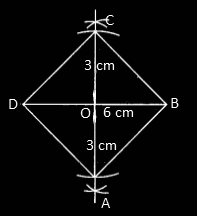### RBSE Maths Chapter 9: Exercise 9.5 Textbook Important Questions and Solutions

Question 31: Construct a trapezium PQRS in which PQ || SR, PQ = 6 cm, RS = 3 cm, PS = 3 cm and QR = 5 cm.

Solution:

Steps of construction:

(i) Draw a line segment PQ of length 6 cm.

(ii) Taking P as the centre, draw an arc of 3 cm and cut line segment PQ at E.

(iii) Now taking E as the centre, draw an arc of 3 cm and also by taking Q as centre draw an arc of 5 cm which intersects the previous arc at R.

(iv) Also, by taking R as the centre, draw an arc of 3 cm and now cut this arc by drawing an arc of 3 cm from P.

Hence, PQRS is the required trapezium.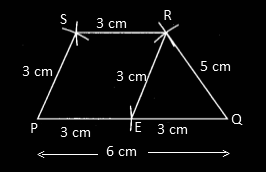Question 32: Construct a trapezium ABCD in which AB || CD, AB = 4 cm, BC = 4 cm, AD = 5 cm and ∠B = 90°.

Solution:

Steps of construction:

(i) Draw a line segment AB of length 4 cm as the base.

(ii) Taking B as the centre, draw an angle line of 90°.

(iii) Now take B as the centre and draw an arc of 4 cm which intersects the angle line at C.

(iv) At C, draw a 90° angle line. With C as the centre, draw an arc of 4 cm which cuts the angle line at E.

(v) Now, by taking A as the centre, draw an arc of 5 cm which will cut the produced line CE at D.

(vi) Join DA, AE.

Hence, ABCD is the required trapezium.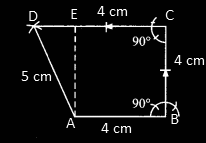### RBSE Maths Chapter 9: Additional Important Questions and Solutions

Question 1: Three angles of a quadrilateral; are 75°, 90°, and 75°. Its fourth angle is:

(a) 90°

(b) 95°

(c) 105°

(d) 120°

Solution:

We know that, the sum of all the interior angles of a quadrilateral = 360°

Let x be the fourth angle.

Thus, 75° + 90° + 75° + x = 360°

240° + x = 360°

x = 360° – 240°

x = 120°

Question 2: A diagonal of a rectangle is inclined at an angle of 25° with a side. The acute angle between its diagonals is:

(a) 55°

(b) 50°

(c) 40°

(d) 25°

Solution:

We know that the diagonals of a rectangle are equal and bisect each other.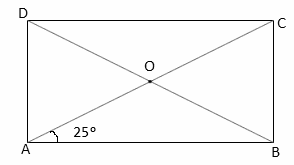In triangle AOB,

∠OAB + ∠ABO + ∠AOB = 180°

25° + 25° + ∠AOB = 180°

∠AOB = 180° – 50° = 130°

DOB is the straight line.

∠AOD + ∠AOB = 180°

∠AOD = 180° – 130° = 50°

Therefore, the acute angle between the diagonals = 50°

Question 3: ABCD is a rhombus in which ∠ACB = 40°. Then ∠ADB is:

(a) 40°

(b) 45°

(c) 50°

(d) 60°

Solution: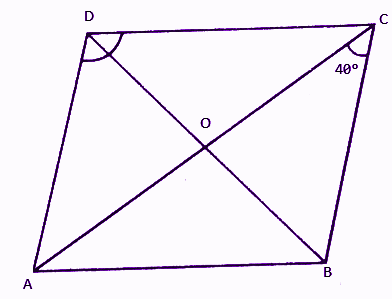In rhombus ABCD,

∠ACB = ∠CAD = 40° (alternate interior angles )

∠AOD = 90° ( diagonal of rhombus bisect each other at 90°)

In triangle AOD ,

∠COD + ∠AOD + ∠ODA = 180°

40° + 90° + ∠ADB = 180°

∠ADB = 180° – 130° = 50°

Question 4: A quadrilateral formed by joining the mid-points of the sides of a quadrilateral PQRS, taken in a order, is a rectangle, if:

(a) PQRS is a rectangle

(b) PQRS is a parallelogram

(c) diagonals of PQRS are perpendicular to each other

(d) diagonals of PQRS are equal

Solution:

According to the given,

Quadrilateral ABCD formed by joining the mid-points of quadrilateral PQRS is a rectangle.

∴ AC = BD (diagonals of a rectangular are equal)

⇒ PQ = QR

Thus, quadrilateral PQRS is a rhombus.

We know that diagonals of rhombus are perpendicular to each other.

Hence, diagonals of PQRS that means PR and QS are perpendicular.

Question 5: If the ratio of angles A, B, C and D of a quadrilateral ABCD, taking in a order is 3 : 7 : 6 : 4, then ABCD is a:

(a) rhombus

(b) parallelogram

(c) trapezium

(d) kite

Solution:

Given ratio of angles: 3 : 7 : 6 : 4

Let 3x, 7x, 6x and 4x be the angles of a quadrilateral.

By the angle sum property of a quadrilateral,

3x + 7x + 6x + 4x = 360°

20x = 360°

x = 360°/20 = 18°

3x = 3(18°) = 54°

7x = 7(18°) = 126°

6x = 6(18°) = 108°

4x = 4(18°) = 72°

Let A = 54°, B = 126°, C = 108°, D = 72°

A + B = 54° + 126° = 180°

C + D = 108° + 72° = 180°

The adjacent angles are supplementary and all the angles are different.

Therefore, the quadrilateral is a trapezium.

Question 6: In quadrilateral ABCD, ∠A + ∠D = 180°. What specific name can be given to this quadrilateral?

Solution:

∠A + ∠D = 180°

That means AB || CD.

Since, the sum of co-interior angles on the same side of traversal is 180°.

Therefore, ABCD is a parallelogram.

Question 7: Any square inside an isosceles right angled is such that one angle is common in both square and triangle. Show that the vertex of the square, which opposite to the vertex of the common angle bisects the hypotenuse.

Solution:

Let ABC be an isosceles right triangle in which ∠A = 90° and AB = AC.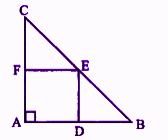and AB = AC ….(ii)

Subtracting (i) from (ii),

AB – AD = AC – AF

⇒ BD = CF ….(iii)

In ∆CFE and ∆EDB,

∠CFE = ∠EDB = 90°

BD = CF (proved above)

DE = EF (sides of a square)

∆CFE = ∆EDB (by SAS congruence criterion)

⇒ CE = BE (by CPCT)

Hence, vertex E of the square bisects the hypotenuse BC.

Question 8: In a parallelogram ABCD, AB = 10 cm and AD = 6 cm. The bisector of ∠A meets DC at E and produce AE and BC to meet at F. Find the length of CF.

Solution: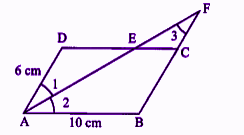∠1 = ∠3 ….(i) (alternate interior angles)

∠1 = ∠2 ….(ii) (AF is the bisector of ∠A)

From (i) and (ii),

∠2 = ∠3

⇒ AB = BF (side opposite to equal angles are equal)

AB = BC + CF

10 = 6 + CF

CF = 4 cm

Question 9: P, Q, R and S are the mid-points of sides AB, BC, CD and DA, respectively in which AC = BD and AC ⊥ BD. Prove that PQRS is a square.

Solution:

Given,

ABCD is a quadrilateral and P, Q, R and S are the mid-points of the side AB, BC, CD and DA respectively.

Also, AC = BD and AC ⊥ BD.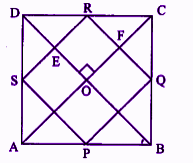Using mid-point theorem,

SR || AC and SR = 1/2 AC ….(i)

In ∆ABC,

PQ || AC and PQ = 1/2 AC ….(ii)

From (i) and (ii),

PQ || SR and PQ = SR ….(iii)

Now, in ∆ABD,

SP || BD and SP = BD = 1/2 AC ….(iv) [since AC = BD (given)]

Inn ∆BCD,

RQ || BD and RQ = BD = 1/2 AC ….(v)

From (iv) and (v),

SP || RQ and SP = RQ = 1/2 AC ….(vi)

From (iii) and (vi),

PQ = SR = SP = RQ

Thus, all four sides are equal.

OE || FR and OF || ER

Thus, OERF is a parallelogram.

∆EOF = ∆ERF = 90° (opposite angles of a parallelogram)

and ∠QRS = 90°

Therefore, PQRS is a square.

Hence proved.

Question 10: E is the mid-point of median AD of ∆ABC. BE is extended to meet AC at F. Show that AF = 1/3 AC.

Solution:

Given,

E is the mid-point of median AD of ∆ABC.

Through D, draw DK || BF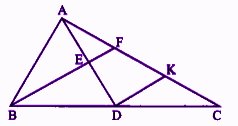E is the mid-point of AD and EF || DK.

F is the mid-point of AK. (by the converse of mid-point theorem)

⇒ AF = FK ….(i)

In ΔBCF,

D is the mid-point of BC and DK || BF.

K is the mid-point of FC.

FK = KC ….(ii)

From (i) and (ii),

AF = FK = KC …(iii)

Now,

AC = AF + FK + KC

AC = AF + AF + AF [(using (iii)]

AC = 3AF

Therefore, AF = 1/3 AC

Hence proved.

Question 11: Construct a rhombus ABCD in which AB = 6 cm and ∠A = 120°.

Solution:

Steps of construction:

(i) Draw a line segment AB of length 6 cm as the base line.

(ii) Taking A as the centre, draw an angle line of 120° and draw an arc with a radius 6 cm and mark the intersection of arc and line as D.

(iii) With B and D as centres, draw arcs with radius 6 cm which intersect each other at C.

(iv) Join BC and CD.

Hence, ABCD is the required trapezium.